# IDL Map Software for Analyzing Solar Images

### 1. INTRODUCTION

An IDL map is a structure that contains two-dimensional (2-d) image data with accompanying pixel coordinate and spatial scale information. The latter parameters are defined as properties of the map and are unique for each image source. Defined in this manner, an arbitrary image can be manipulated or transformed in a manner that is independent of the image source.

This software note describes how to create and use maps for processing solar images. We will use sample images obtained from instruments onboard SOHO and Yohkoh missions. Typical processing tasks include: roll-correction, stretching, translation, solar rotation-compensation, and image coalignment. Although we discuss solar examples, the techniques described below are applicable to any two-dimensional dataset. IDL mapping software is incorporated into the SolarSoft system - a set of integrated software libraries, databases, and system utilities which provide a "common" programming and data analysis environment for Solar Physics.

### 1.1 Creating a Map

The low-level function for creating a map is make_map. In this example, a 256x256 array is created with findgen and converted to a map variable: ``` ```

``````IDL> image=findgen(256,256)
IDL> map=make_map(image)
IDL> help,/st,map

** Structure <4031f908>, 8 tags, length=40056, refs=1:
DATA            FLOAT     Array[256,256]
XC              FLOAT           0.00000
YC              FLOAT           0.00000
DX              FLOAT           1.00000
DY              FLOAT           1.00000
TIME            STRING    '11-Mar-1998 11:40:44.000'
``````
``` ``` The resulting map is an anonymous structure with the following tag definitions:
• DATA: the 2-d data values.
• XC, YC: the cartesian coordinates of center of the image. Since coordinate information was not specified, the origin is used as the default value.
• DX, DY: the spacing between between pixels in the cartesian X and Y directions, respectively. Since spatial scale was not specified, the spacings default to unity.
• TIME: a reference time for the image. It could be the start or mean time of the image. The default time is the current Universal Time (UT) when the map is created.

The above tag definitions are defined also as keywords in make_map. For example, a map with a pixel spacing of 2 units in the x-direction and 3 units in the y-direction is created by:

``````IDL> image=findgen(256,256)
IDL> map=make_map(image,dx=2,dy=3)
IDL> help,/st,map
** Structure <403cd0e8>, 9 tags, length=40072, refs=1:
DATA            FLOAT     Array[100, 100]
XC              FLOAT           0.00000
YC              FLOAT           0.00000
DX              FLOAT           2.00000
DY              FLOAT           3.00000
TIME            STRING    '18-Mar-1998 15:23:16.000'
``````

Note that the basic map structure definition is kept intentionally simple, with data and coordinate parameters being the main defining properties. The units of the coordinate system are also arbitrary. Additional properties are added two ways:

1. At the definition stage. For example, to include a property UNITS that specifies coordinate units:

``````IDL> nmap=make_map(map,units='arcsecs')
IDL> help,nmap,/st
** Structure <403cd0e8>, 9 tags, length=40072, refs=1:
DATA            FLOAT     Array[100, 100]
XC              FLOAT           0.00000
YC              FLOAT           0.00000
DX              FLOAT           2.00000
DY              FLOAT           3.00000
TIME            STRING    '18-Mar-1998 15:23:16.000'
UNITS           STRING    'arcsecs'
``````
2. After the definition stage. Following the same example, but using the function add_prop :

``````

``````

produces the same result. Multiple properties are added as multiple keywords, with the restriction that each property name is unique.

### 1.2 Plotting a Map

Maps are plotted using the procedure plot_map . Thus, using the 2-d image created earlier,

``````IDL> plot_map,map
``````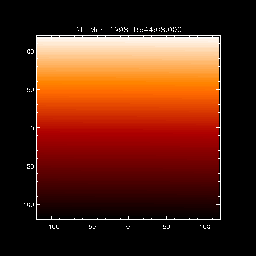Fig. 1 - Image Map

The procedure plot_map inherits all the major IDL plot keywords such as xstyle, ystyle, font, charsize, charthick, etc. It supports the following additional keywords:

• drange: 2-element vector of data min and max to display
• xrange: 2-element vector of xmin and xmax to display
• yrange: 2-element vector of ymin and ymax to display
• /log: display using log10 scaling
• xsize, ysize: device pixel size for the plot window [def=512x512]
• /noaxes: inhibit plotting axes
• /nolabels: inhibit printing axis labels
• /notitle: inhibit printing title
• /cont: plot image as a contour
• levels: an array of contour levels

The use of more advanced keywords will be demonstrated later in this tutorial.

### 1.3 Plotting Maps on Different Color Scales

The following steps demonstrate how to simultaneously plot maps using different color tables.

``````
IDL> image=findgen(256,256)
IDL> map=make_map(image)                ;-- make a simple map
IDL> nc=100                             ;-- # of colors per image
IDL> plot_map,map,ncolors=nc            ;-- plot map using red table
IDL> plot_map,map,bottom=nc,ncolors=nc  ;-- plot using blue table

``````

The key to plotting with separate color tables is to divide evenly the total number of available device colors. The latter is given by the system variable !d.table_size. In the above example, the total available colors is 200. Hence, the color table is split into two equal halves of nc=100 colors each. The routine loadct loads a red color table (#3) into the first 0 to nc-1 color range, and blue color table (#1) into the second nc to 2*nc-1 range. The resulting plots appear as:

### 2.1 Rotating a Map

Maps are rotated using the function rot_map . An interesting example is provided by rotating (i.e, rolling) a SOHO EIT full Sun image. The routines for creating an EIT map will be described in section 3.1 . For now, assume a 512x512 EIT map, eit_map with the following properties:

``````
DATA            INT       Array[512, 512]
XC              FLOAT           14.2450
YC              FLOAT          -13.0278
DX              FLOAT           5.18000
DY              FLOAT           5.18000
TIME            STRING    '15-Mar-1998 00:00:49.985'
DUR             FLOAT           12.1000
ID              STRING    'EIT: 304'
SOHO            INT              1
UNITS           STRING    'arcsecs'

``````

This map contains an EIT 304 Angstrom image that has been double-binned from its original 1024x1024 size. Note the additional properties ID specifying the image wavelength, DUR specifying the image duration, and SOHO specifying image source. To rotate this map through, say, 60 degrees clockwise about its center, and display the results:

``````
IDL> rmap=rot_map(eit_map,60.)
IDL> plot_map,eit_map,/log
IDL> plot_map,rmap,/log,/new

IDL> help,/st,rmap

DATA            INT       Array[512, 512]
XC              FLOAT           14.2450
YC              FLOAT          -13.0278
DX              FLOAT           5.18000
DY              FLOAT           5.18000
TIME            STRING    '15-Mar-1998 00:00:49.985'
DUR             FLOAT           12.1000
ROLL            FLOAT           60.0000
ROLL_CENTER     FLOAT     Array
ID              STRING    'EIT: 304'
SOHO            INT              1
UNITS           STRING    'arcsecs'

``````

The keyword /new forces the creation of a new plot window in which to plot the rotated image. The rotation is performed by rotating the coordinates of each image pixel, computing a new grid of rotated coordinates, and interpolating the new grid back to the original image. Generally, the dimensions of the rotated grid will be larger than the original image. By default, rot_map will preserve the original image dimensions by clipping image pixels that fall outside the original dimensions. The keyword /full_size inhibits clipping.

The roll angle and roll center are added as property fields name ROLL and ROLL_CENTER to the rotated map. The roll angle in units of degrees measured clockwise from North and the roll center coordinates are in data units (i.e., arcsecs). Note that if the input map is already rolled, then the new roll angle will be added to the existing roll value. By default, the map is rotated about its center. The keyword center can be used to rotate about an arbitrary center, e.g., center=[20,30] .

The keyword roll has the same functionality and can be used to roll a map to any given roll angle, regardless of its current roll angle. For example, to rotate a map to a 100 degree roll:

``````
IDL> rmap=rot_map(eit_map,roll=100.)

``````

### 2.2 Stretching and Resizing a Map

The function grid_map changes the dimensions and pixel spacing of a map. For example, the following command will rebin the 512x512 EIT map displayed in figure 3a, to a 128x128 size map:

``````
IDL> gmap=grid_map(map,128,128)

``````

The (x,y) pixel spacings of the output map are computed automatically. To change the spacing between pixels, use the keywords dx, dy . For example, to rebin to a pixel spacing of 2 units in x- and 5 in y-directions:

``````
IDL> gmap=grid_map(map,dx=2,dy=5)

``````

The (x,y) dimensions of the output map are computed automatically. Spacing and output dimension parameters can be combined to produce different stretching and resizing effects. For large images, regridding can be a slow process since each pixel has to be interpolated onto a new spatial scale.

### 2.3 Extracting a Sub-Region From a Map

The procedure sub_map extracts a sub-region from a map. It can be used in two ways, graphically or manually.

``````
IDL> sub_map,map,smap,/plot,[xrange=xrange,yrange=yrange,ref_map=ref_map]

``````

A sub-region of map is selected graphically by using the keyword /plot, in which case plot_map is called and a "rubber-band" cursor is used to select a sub-region. The sub-region map is returned in smap. The data coordinate limits of the sub-region are returned as two-element vectors in the keywords xrange and yrange. Alternatively, the data coordinate limits can be input via the keywords xrange=[xmin,xmax], yrange=[ymin,ymax], or via the keyword ref_map. In the latter case, the extracted sub-region will be extracted according to the xrange/yrange values of ref_map.

### 2.4 Differencing Maps

The procedure diff_map subtracts two maps to produce a difference map.

``````
IDL> dmap=diff_map(map1,map2,[/rotate])

``````

The output map dmap contains a difference image of the two images contained in the map1 and map2 input maps -- the second image is subtracted from the first. The optional keyword /rotate solar rotates the second image to the time of the first image prior to subtraction. Solar rotation is described in section 3.6 .

### 3. APPLYING MAPS TO SOLAR IMAGES

This section describes the steps involved in creating maps for various solar datasets. The routine index2map is a generally useful program for creating maps from any dataset that is in a Yohkoh index/data format.

### 3.1 Creating a SOHO/EIT Map

The following lines read and process EIT images into maps:

``````
IDL> files=eit_files('19-mar-98','20-mar-98',wave=304,/full)
IDL> eit_prep,index,data=data,outindex,outdata
IDL> index2map,outindex,outdata,emap

``````

Each step is summarized as follows:

1. eit_files returns EIT filenames for a range of times/dates and wavelength filter. This example returns filenames (string array) for 304 Angstom full-disk images.
2. read_eit reads the filenames array and returns an index structure representation of the FITS file header and a 2-d data array of image values. The latter is a 3-d array when more than one image is read. The optional keyword outsize specifies the dimensions of the output image. For example, setting outsize=512 produces a 512x512 image.
3. eit_prep applies degridding, dark current, and flatfield corrections to the image data.
4. index2map converts the corrected outindex and outdata variables into a map. The latter is an array when multiple images are copied.

In the case of full-disk images, the 1024x1024 array size can lead to IDL out-of-memory problems on some systems. To avoid this problem, there is an optional outsize keyword to rebin images to a smaller dimension (with accompanying loss of spatial resolution). The following example creates a 512x512 map.

``````
IDL>index2map,outindex,outdata,emap,outsize=512

``````

The outsize keyword can also be used when reading the image with read_eit. More detailed documentation on manipulating EIT data is located in the EIT Users Guide .

### 3.2 Creating a SOHO/CDS Map

The function mk_cds_map creates CDS maps. The CDS instrument produces images by rastering in pre-determined spectral lines. Images can be derived by using the peak count rate intensity of these lines, or by integrating the count rates over the observed spectral wavelength band. The following steps illustrate reading and processing CDS images into maps:

``````
IDL> files=cds_files('19-mar-98','20-mar-98',study=study,info=info)
IDL> cds_map=mk_cds_map(ql,window,/calib,/clean)

``````

Each step is summarized as follows:

1. cds_files returns CDS filenames for a range of times/dates. This procedure requires that the environment variable CDS_FITS_DATA point to the directory containing the CDS FITS files. An optional input keyword study can be used to search for specific files. This keyword is an acronym for the CDS study, e.g. study='SYNOP' . An optional output keyword info returns a string array of observation start times and study names for the files. Currently, the mapping software requires that only maps with similar dimensions can be combined. Since CDS studies can have arbitrary raster sizes, one must be careful not to mix different studies into an array of maps.
2. read_cds reads each file name and returns an array of "quicklook" data structures QL. Each QL structure contains a single raster experiment in several simultaneous wavelengths.
3. mk_cds_map creates maps for selected selected spectral windows. The window argument is a scalar or vector of window numbers. Each window corresponds to a particular wavelength range. If this argument is not included, then the function will prompt for window numbers. The following keywords are also supported:
• /calib: applies instrumental corrections for background, flatfield, and spectral tilt.
• /clean: removes data pixels corrupted by cosmic ray hits.
• /peak: produces an image based on the peak intensity within the window spectral band -- default behavior is to sum over wavelength.

### 3.3 Creating a Yohkoh/SXT Map

The following example, reads and creates an SXT map from an SXT full-frame data file:

``` ```

``````IDL> rd_xda,'sfr970407.1229',-1,index,data
IDL> sxt_prep,index,data,outindex,outdata,/register,/norm,/roll
IDL> index2map,outindex,outdata,sxt_map
``````
``` ```

Details on the use of rd_xda for reading SXT files can be found in the Yohkoh Analysis Guide. The procedure sxt_prep applies instrumental corrections to the SXT raw image that include (among other things) adjustment for spacecraft jitter, roll, dark current subtraction, and exposure normalization. Multiple images can be combined with the restriction that they have the same dimensions. Figure 4 shows a map created from an SXT full-frame AlMg filter image with the following properties:

``````
IDL> help,/st,sxt_map
** Structure <40a74fe8>, 9 tags, length=1048648, refs=2:
DATA            FLOAT     Array[512, 512]
XC              FLOAT          -73.8006
YC              FLOAT          -154.193
DX              FLOAT           4.91000
DY              FLOAT           4.91000
TIME            STRING    ' 6-Jun-1996 19:30:37.000'
DUR             FLOAT           1.00000
ID              STRING    'AlMg '
UNITS           STRING    'arcsecs'

IDL> plot_map,sxt_map,/log,grid=20

``````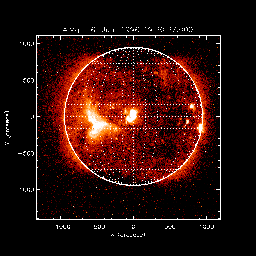Fig. 4 - SXT map

The above image is plotted using the keyword grid=20. This keyword enables overlaying of a latitude and longitude grid with a grid spacing equal to the keyword value in units of degrees. Setting grid to a negative value will disable gridding, but will enable plotting of the optical solar limb. The same effect is achieved using the keyword /limb.

### 3.4 Converting a TRACE File to a Map

TRACE files are read and converted to maps as follows.

``````
IDL> index2map,index,data,trace_map
IDL> plot_map,trace_map

``````

where the procedure read_trace is a special purpose reader for TRACE files.

### 3.5 Converting a FITS File to a Map

FITS files can be converted to maps by using the procedure fits2map . The following example reads and converts a SOHO/MDI full-disk magnetogram FITS file into a map, and plots the result:

``````
IDL> plot_map,map

``````

The keyword header optionally returns the string header of the FITS file.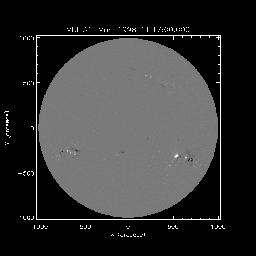Fig. 5 - MDI map

### 3.5.1 Converting a RHESSI FITS File to a Map

RHESSI FITS files are converted to maps by using fits2map. RHESSI images can be created using the RHESSI GUI and saved as FITS files

### 3.6 Correcting for Solar Differential Rotation

The function drot_map rotates a map using the solar differential rotation formula of Howard, Harvey, and Forgach, Solar Physics, 130, 295, 1990. Since the formula is derived from statistical studies of small-scale magnetic features, it is most accurately applied to photospheric images and is less accurate when applied to transition region and coronal images observed by EIT and SXT, respectively. The following example demonstrates the differential rotation of the above full-disk SXT image over 4 days.

``````
IDL> gmap=grid_map(sxt_map,256,256)
IDL> dmap=drot_map(gmap,4,/days)
IDL> plot_map,sxt_map,/log
IDL> plot_map,dmap,/log,/new,/limb

``````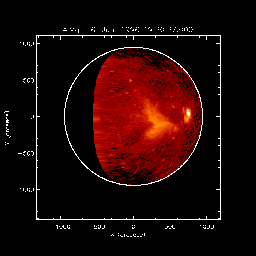Fig. 6 - Rotated SXT map

Because solar rotation involves interpolating each pixel to a new location, processing large 512x512 or 1024x1024 images can be very time-consuming and memory-intensive on some systems. In the above example, the SXT map is rebinned to a more manageable 256x256 size prior to rotation. The function drot_map accepts map and duration (in hours) as input arguments. It also supports the following keywords:

• /days: specifies that input duration is in units of days.
• /secs: specifies that input duration is in units of seconds.
• time=new_time: specifies the new time to which the image is to be rotated. In this case, the duration argument should not be used (since it always takes precedence).
• missing=value: specifies the value assigned to pixels that have rotated out of the field or over the limb -- the default is 0.

The function drot_map combines regridding, roll-correction, and translation through the following additional keywords:

• space=[dx,dy]: specifies new pixel spacings.
• roll=new_roll: specifies new roll angle (in degrees).
• center = [rx,ry]: specifies new roll center.
• trans = [xs,ys]: specifies translation shifts in the x- and y- directions.
• ncenter = [nx,ny]: specifies a new center coordinate for the solar-rotated image.
• ref_map=ref_map: specifies a reference map to which the input map is to be mapped (i.e, use reference map time, spacing, roll, center, ncenter, etc).

### 4. OVERLAYING MAPS

Maps are graphically overlayed using the keyword /over in plot_map . In the following example, an SXT map is contoured over an EIT map.

``````
IDL>plot_map,eit_map,fov=sxt_map,/log  ;-- first plot EIT
IDL>plot_map,sxt_map,/log,/over,/rotate  ;-- then contour over SXT

``````

The fov keyword is a map structure from which plot_map infers xrange and yrange values. In the above example, only the sub-region of eit_map that overlaps with that of sxt_map field-of-view is displayed. This keyword is optional. Different sub-regions can be displayed also via the xrange and yrange keywords.

The /rotate keyword corrects for solar rotation of the overlayed image relative to the first. This correction is important if the time difference between images is more than several hours. The overlayed image can be rotated to a specified time via the keyword time.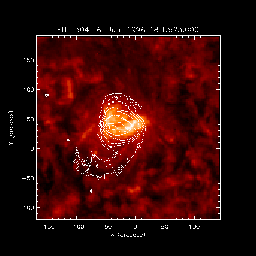Fig. 7c - SXT/EIT Overlay

The following example shows an overlay of MDI magnetogram contours on an SXT observation of flare loops. The example illustrates the use of additional keyword options in plot_map.Fig. 8 - MDI/SXT Overlay

``````
IDL>set_line_color
IDL>levels=100+findgen(11)*100.
IDL>plot_map,sxt_map,/log,bottom=11
IDL>plot_map,mdi_map,/over,/rotate,/positive,c_color=5,levels=levels
IDL>plot_map,mdi_map,/over,/rotate,/negative,c_color=2,levels=-levels

``````

Each step is explained below:

1. set_line_color allocates a simple color table between 0 and 10, in which color=2 corresponds to yellow, and color=5 corresponds to blue. When using set_line_color, it is necessary that plot_map be called with the keyword: bottom=11 to ensure that the image data are scaled to the correct number of available colors above the 11 colors reserved by set_line_color.
2. findgen is used to define an array of contour levels: 100,200...1000.
3. the SXT map specified in sxt_map is plotted.
4. positive MDI magnetogram values in the map mdi_map are overplotted as blue contours.
5. negative MDI magnetogram values are overplotted as yellow contours.

The final example shows an overlay of a CDS Ne VI map on an EIT 171 map, for a limb active region.

``````
IDL>plot_map,eit_map,/log
IDL>plot_map,cds_map,/over,cthick=2

``````

The keyword cthick=2 specifies a double thickness contour for the overlay image.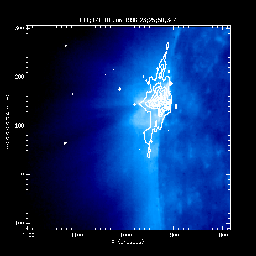Fig. 9c - CDS/EIT Overlay

Last Revised: